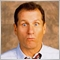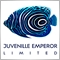# Help modifying an Indicator49

Hello all,

I could need some help with this modification. I am trying to change the OBJ_VLINE to a OBJ_TREND in order to stop it from drawing the line on the bottom indicator window and only drawing the line on the chart. But I am unsuccesful as my coding skills are basic at best.

Any help would be greatly appreciated.

This is the Indicator, source is ForexFactory

```//+------------------------------------------------------------------+
//|                                                  Mn TimeLine.mq4 |
//+------------------------------------------------------------------+

#property indicator_chart_window
extern color mCol = DimGray;
extern int mStyle = 3, mWidth = 0,
mH1 = 7,  mM1 = 30,
mH2 = 8,  mM2 = 30,
mH3 = 12,  mM3 = 30,
mH4 = 13,  mM4 = 30,
mH5 = 20,  mM5 = 30,
mH6 = 21,  mM6 = 30,
mH7 = 20,  mM7 = 30,
mH8 = 21,  mM8 = 30,
mH9 = 7,  mM9 = 30,
mH10 = 80,  mM10 = 30;

int mTimes,
mDay,
mTimeBar;

//+------------------------------------------------------------------+
int init()
{

return(0);
}
//+------------------------------------------------------------------+
int deinit()
{
for(int y = ObjectsTotal(); y >= 0; y--)
{
if(StringSubstr(ObjectName(y), 0, 3) == "mVL")
ObjectDelete(ObjectName(y));
}

return(0);
}
//+------------------------------------------------------------------+
int start()
{
if(iTime(NULL, 1440, 0) > mTimeBar)
{
for(int i = 0; i < 20; i ++)
{
mDay[i]  = iTime(NULL, 1440, i);
mTimes[i] = mH1 * 3600 + mM1 * 60 + mDay[i];
mTimes[i] = mH2 * 3600 + mM2 * 60 + mDay[i];
mTimes[i] = mH3 * 3600 + mM3 * 60 + mDay[i];
mTimes[i] = mH4 * 3600 + mM4 * 60 + mDay[i];
mTimes[i] = mH5 * 3600 + mM5 * 60 + mDay[i];
mTimes[i] = mH6 * 3600 + mM6 * 60 + mDay[i];
mTimes[i] = mH7 * 3600 + mM7 * 60 + mDay[i];
mTimes[i] = mH8 * 3600 + mM8 * 60 + mDay[i];
mTimes[i] = mH9 * 3600 + mM9 * 60 + mDay[i];
mTimes[i] = mH10 * 3600 + mM10 * 60 + mDay[i];

for(int j = 0; j < ArraySize(mTimes); j++)
{
if(ObjectFind("mVL"+ mTimes[j][i]) == -1)
ObjectCreate("mVL"+ mTimes[j][i], OBJ_VLINE, 0, 0, 0, 0, 0);
ObjectSet("mVL"+ mTimes[j][i], OBJPROP_TIME1 , mTimes[j][i]);
ObjectSet("mVL"+ mTimes[j][i], OBJPROP_COLOR , mCol);
ObjectSet("mVL"+ mTimes[j][i], OBJPROP_STYLE , mStyle);
ObjectSet("mVL"+ mTimes[j][i], OBJPROP_WIDTH, mWidth);
ObjectSet("mVL"+ mTimes[j][i], OBJPROP_BACK, true);
}
}
mTimeBar = iTime(NULL, 1440, 0);
}

return(0);
}
//+------------------------------------------------------------------+```

Many thanks

Files:Moderator
10225

Use the </> button to insert your code.49

Well, managed it on my own in the end. These are the changes I made:

```if(ObjectFind("mVL"+ mTimes[j][i]) == -1)
ObjectCreate("mVL"+ mTimes[j][i], OBJ_TREND, 0, 0, 0, 0, 0);
ObjectSet("mVL"+ mTimes[j][i], OBJPROP_TIME1 , mTimes[j][i]);
ObjectSet("mVL"+ mTimes[j][i], OBJPROP_TIME2 , mTimes[j][i]);
ObjectSet("mVL"+ mTimes[j][i], OBJPROP_PRICE1 , 500000);
ObjectSet("mVL"+ mTimes[j][i], OBJPROP_PRICE2 , 0);
ObjectSet("mVL"+ mTimes[j][i], OBJPROP_COLOR , mCol);
ObjectSet("mVL"+ mTimes[j][i], OBJPROP_STYLE , mStyle);
ObjectSet("mVL"+ mTimes[j][i], OBJPROP_WIDTH, mWidth);
ObjectSet("mVL"+ mTimes[j][i], OBJPROP_BACK, true);```

Works well so far